# Class 12 Board Exams - Top Maths Formulae You Should Know

###### ByRamraj Saini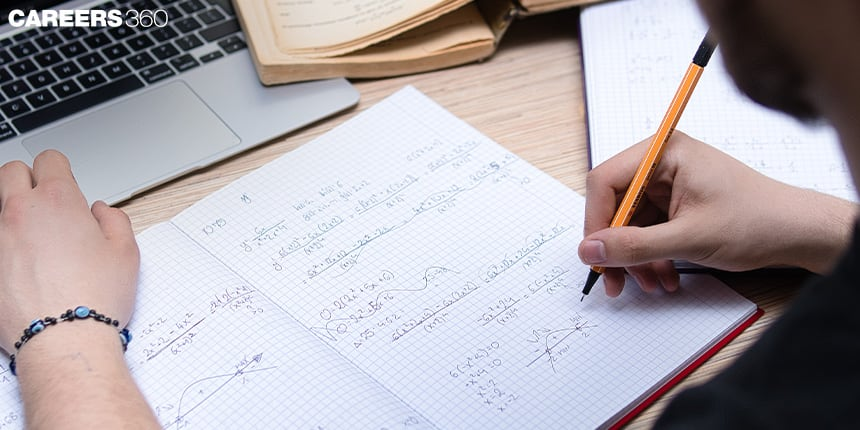###### Synopsis

There is often a fear of Maths among students. Especially as exams approach, the subject becomes somewhat of a nightmare. But mastering mathematical formulas can help relieve you of this fear. In this article Careers360 has listed concept-wise and chapter-wise Maths formulas specially for Class 12 students.###### Synopsis

There is often a fear of Maths among students. Especially as exams approach, the subject becomes somewhat of a nightmare. But mastering mathematical formulas can help relieve you of this fear. In this article Careers360 has listed concept-wise and chapter-wise Maths formulas specially for Class 12 students.

Learning the various mathematics formulas is the only way you can get rid of their fear of the subject. Students can achieve the marks they desire if they can master learning the mathematics formula. Understanding the concepts, learning the formulas and finally applying these formulas to find the solution is all that is required for you to do.

Once students can grasp the various mathematical formulas it becomes very easy to find the solution to any particular problem. Moreover, it allows you to solve problems faster. So, to make it easier for yourself, make a note of important mathematical formulas and keep them handy so that you can keep revising them till you give your exam.

Here is a list of important Mathematics formulas for the board exam Class 12.

## Chapter 2: Inverse Trigonometric Functions

Some definitions

• y= sin-1 (x) => x= sin(y)

• y = cos-1 (x) => x= cos(y)

• y= tan-1 (x) => x= tan(y)

Important inverse properties

sin ( sin-1 (x) ) = x , cos ( cos-1 (x) ) = x , tan ( tan-1 (x) ) = x

sin-1 (sin (x) ) = x , cos-1 (cos (x) ) = x , tan-1 (tan (x) ) = x

sin-1 (-x) = - sin-1 (x) and cosec-1 (-x) = - cosec-1 (x)

cos-1 (- x) = π - cos-1 (x) and sec-1 (- x) = π - sec-1 (x)

tan-1 (- x) = - tan-1 (x) and cot-1 (- x) = π - cot-1 (x)

sin-1 (x) + cos-1 (x) = π/2

tan-1 (x) + cot-1 (x) = π/2

sec-1 (x) + cosec-1 (x) = π/2

tan -1 (x) + tan -1 (y) = tan -1 (x) {(x + y)/ (1- xy)}

2 tan -1 (x) = sin-1 {(2x)/( 1 + x2)} = cos-1 { (1- x2 )/ ( 1 + x2)}

2 tan -1 (x) = tan -1 {(2x) / (1- x2 )}

tan -1 (x) + tan -1 (y) = π + tan -1 {(x + y) /( 1- xy)} , xy > 1 ; x , y > 0

## Chapter 3 And 4: Matrix And Determinant

Definitions and theorems:

• An m × n matrix will be a square matrix if m = n.

• A zero matrix will contain all its elements as zero.

• A = [aij]m × m will be a diagonal matrix if aij = 0, when i ≠ j.

• A = [aij]n × n will be an identity matrix, if aij = 1, when i = j and aij= 0, when i ≠ j.

• A = [aij]n × n will be a scalar matrix if aij= 0, when i ≠ j, aij = k,

(where k = constant); and i = j.

• A = [aij] = [bij] = B if and only if: A and B are of the same order, aij = bij for all the certain values of i and j.

Some basic operations:

• kA = k[aij]m × n = [k(aij)]m × n

• – A = (– 1)A

• A – B = A + (– 1)B

• A + B = B + A

• (A + B) + C = A + (B + C); where A, B, and C all are of the same order

• k(A + B) = kA + kB; where A and B are of the same order and k = constant

• (k + l)A = kA + lA; where k and l are constants.

• If A= [aij]m × n, then A′ or AT = [aji]n × m

• (A′)′ = A

• (kA)′ = kA′

• (A + B)′ = A′ +B′

• (AB)′ = B′A′

Some elementary operations:

• Ri ↔ Rj or Ci ↔ Cj

• Ri→ kRi or Ci → kCi

• Ri →Ri + kRj or Ci→ Ci + kCj

A is known as a symmetric matrix if A′ = A

A is known as a skew-symmetric matrix if A′ = –A

For a square matrix A for equation AX = B

• |A| ≠ 0, a unique solution exists

• |A| = 0 and (adj A) B ≠ 0, no solution exists

• |A| = 0 and (adj A) B = 0, the system may or may not be consistent.

## Chapter 5: Continuity And Differentiability

• A function will be a continuous function if the limit of that function at a given point is equal to the value of the function at the same point.

• Properties related to the functions:

• (f ± g)(x) = f(x) ± g(x) is continuous.

• (f.g)(x) = f(x).g(x) is continuous.

• (f / g)(x) = f(x) / g(x) ( whenever g(x) ≠ 0 is continuous ).

• Chain Rule: If f = v o u, t = u (x) and if both

dt/dx and dv/dx exist, then (df/dx) = (dv/dt).(dt/dx)

• Rolle’s Theorem: If f: [a, b] → R is continuous on [a, b], differentiable on (a, b) whereas f(a) = f(b), then there exists some c in (a, b) such that f ′(c) = 0.

• Mean Value Theorem: If f : [a, b] → R is continuous on [a, b] and differentiable on (a, b). Then there exists some c in (a, b) such that

f′(c) = (f(b) - f(a)) / (b - a)

## Chapters 7 And 8: Integrals And Their Applications

• Integration is the inverse process of differentiation. Suppose,

d/dx{F(x)} = f(x) then we can write ∫ f(x)dx=F(x)+C

• Properties of indefinite integrals:

∫ [f(x)+g(x)] dx = ∫f(x)dx+∫g(x)dx

• For any real number k, ∫kf(x)dx = k∫f(x)dx

• ∫ xn dx = ( xn+1 ) / (n +1), n ≠ −1, Particularly, ∫dx = x + C

• ∫ cosec(x).cot(x) dx = -cosec(x) + C

• ∫ sec(x).tan(x) dx = sec(x) + C

• ∫ cosec2(x) dx = - cot(x) + C

• ∫ sec2(x) dx = tan(x) + C

• ∫ sin(x) dx = cos(x) + C

• ∫ cos(x) dx = - sin(x) + C

• ∫ tan(x) dx = log |sec(x)| + C

• ∫ cot(x) dx = log|sin(x)| + C

• ∫ sec(x) dx = log| sec(x) + tan(x) | + C

• ∫ cosec(x) dx = log| cosec(x) - cot(x) | + C

## Chapter 9: Differential Equation

Differential equations are a type of mathematical equation that involves derivatives of one or more functions. Some important formulae in the differential equation include

• The general form of a first-order differential equation is given by: dy/dx = f(x, y), where y is the dependent variable and x is the independent variable.

• The general form of a second-order differential equation is given by: d2y/dx2 + p(x)dy/dx + q(x)y = r(x), where y is the dependent variable, x is the independent variable, and p(x), q(x), and r(x) are functions of x.

• The solution to a first-order differential equation can be found using the formula: y = y0 + ∫f(x, y)dx, where y0 is the initial value of y at some point x0 and ∫f(x, y)dx is the indefinite integral of f(x, y) with respect to x.

• The solution to a second-order differential equation can be found using the formula: y = c1y1(x) + c2y2(x), where y1(x) and y2(x) are two linearly independent solutions to the equation and c1 and c2 are constants that can be determined by the initial conditions.

Following are some other important formulas listed below.

xdy + ydx = d(xy)

d(x+y) = dx + dy

d(y/x) = (xdy-ydx)/x2

(xdy + ydx)/xy = d(log xy)

(xdy - ydx)/xy = d(logx/y)

(xdy + ydx)/(x2 + y2)= d(log(√(x2+y2))

(xdy - ydx)/(x2 + y2)=d(tan-1(x/y))

d(ex/y)= (yexdy - exdy)/y2

## Chapter 10 And 11: Vector algebra And Three-dimensional Geometry

• The scalar product of two given vectors a and b having angle θ between them is.

a . b = |a||b | cos(θ )

• Vector equation of a line that passes through a vector a and parallel to the vector b is,

r = a + λb

• The vector equation of a line which passes through two points whose position vectors a and b

r = a + λ( b - a )

• The position vector of a point R dividing a line segment joining the points P and Q whose position vectors a and b respectively in the ratio m : n is given by:

(i) Internally = (na + mb)/(m + n)

(ii) Externally = (na - mb )/ (m - n)

• The distance between parallel lines r = a1 + λb1 and

r = a2 + μ b2

(|(b1⨯b2).( a2 - a1) | )/ (|(b1⨯b2)|)

• If l, m, and n are the direction cosines of a line, then l2 + m2 + n2= 1.

• The Direction cosines of a line joining two points P (x1, y1, z1) and

Q (x2, y2, z2 ) are,

(x2- x1)/PQ, (y2 - y1)/ PQ , (z2- z1)/PQ

Where, PQ = √((x2-x1)2+(y2-y1)2+(z2-z1)2)

• Equation of a line which is passing through a point (x1 , y1 , z1 ), having direction cosines l, m, n is:

(x - x1) / l = (y - y1) / m = (z - z1) / n

• Equation of a plane which is passing through a given point (x1 , y1 , z1 ) and perpendicular to a given line with direction ratios P, Q, R and is,

P (x - x1) + Q (y - y1)+ R (z - z1)= 0

• If l, m, n are direction cosines and a,b,c are directions ratios of a line

l = a/(√a2 + b2 + c2); m = b/(√a2 + b2 + c2); n = c/(√a2 + b2 + c2)

• Cartesian equation of a line which is passing through two points (x1,y1,z1) and (x2,y2,z2) is,

(x-x1)/(x2 - x1) = (y-y1)/(y2 - y1) = (z-z1)/(z2 - z1)

## Chapter 13: Probability

There are several important formulas in probability theory that are used to calculate the likelihood of different events occurring. Some of the most important ones include:

• The probability of an event occurring is given by the formula:

P(A) = n(A) / n(S),

Where, P(A) is the probability of the event,

n(A) is the number of favorable events that occur,

n(S) is the total number of possible outcomes.

• The probability of two independent events:

P(A ∩ B) = P(A) * P(B),

Where P(A) and P(B) are the probabilities of both events occurring.

• The probability of an event occurring given that another event has already occurred is called conditional probability and is given by the formula:

P(A | B) = P(A ∩ B) / P(B),

Where, P(A ∩ B) is the probability of both events occurring

P(B) is the probability of the second event occurring.

• P(A ∪ B) = P(A) + P(B) - P(A∩B)

• The probability of an event not occurring is given by the formula:

P(not A) = 1 - P(A),

Where P(A) is the probability of the event occurring.

• Theorem of total probability:

Let S be a sample space and let A1 , A2, ...,An be a partition of S,

i.e. the events A1, A2, ..., An are disjoint and their union is S. Let B be an event in S. Then the probability of B occurring is given by:

P(B) = P(B | A1 ) * P(A1 ) + P(B | A2) * P(A2) + ... + P(B | An) * P(An)

Understanding these formulae and being able to apply them correctly will help you to solve problems effectively and accurately, which is crucial for success in your exams and in your future studies and career.

## Careers360 helping shape your Career for a better tomorrow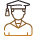#### 250M+

Students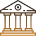#### 30,000+

Colleges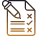#### 500+

Exams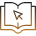E-Books